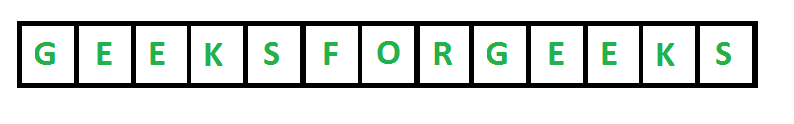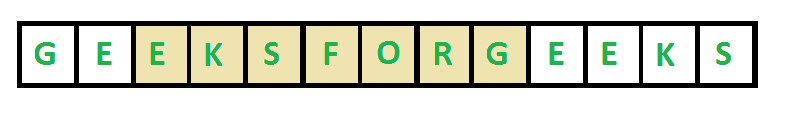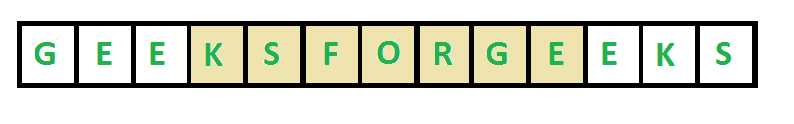# Python Program To Find Length Of The Longest Substring Without Repeating Characters

• Last Updated : 20 Dec, 2021

Given a string str, find the length of the longest substring without repeating characters.

• For “ABDEFGABEF”, the longest substring are “BDEFGA” and “DEFGAB”, with length 6.
• For “BBBB” the longest substring is “B”, with length 1.
• For “GEEKSFORGEEKS”, there are two longest substrings shown in the below diagrams, with length 7The desired time complexity is O(n) where n is the length of the string.

Method 1 (Simple : O(n3)): We can consider all substrings one by one and check for each substring whether it contains all unique characters or not. There will be n*(n+1)/2 substrings. Whether a substring contains all unique characters or not can be checked in linear time by scanning it from left to right and keeping a map of visited characters. Time complexity of this solution would be O(n^3).

## Python3

 `# Python3 program to find the length``# of the longest substring without``# repeating characters`` ` `# This functionr eturns true if all``# characters in strr[i..j] are ``# distinct, otherwise returns false``def` `areDistinct(strr, i, j):`` ` `    ``# Note : Default values in visited are false``    ``visited ``=` `[``0``] ``*` `(``26``)`` ` `    ``for` `k ``in` `range``(i, j ``+` `1``):``        ``if` `(visited[``ord``(strr[k]) ``-` `                   ``ord``(``'a'``)] ``=``=` `True``):``            ``return` `False``             ` `        ``visited[``ord``(strr[k]) ``-``                ``ord``(``'a'``)] ``=` `True`` ` `    ``return` `True`` ` `# Returns length of the longest substring``# with all distinct characters.``def` `longestUniqueSubsttr(strr):``     ` `    ``n ``=` `len``(strr)``     ` `    ``# Result``    ``res ``=` `0` `     ` `    ``for` `i ``in` `range``(n):``        ``for` `j ``in` `range``(i, n):``            ``if` `(areDistinct(strr, i, j)):``                ``res ``=` `max``(res, j ``-` `i ``+` `1``)``                 ` `    ``return` `res`` ` `# Driver code``if` `__name__ ``=``=` `'__main__'``:``     ` `    ``strr ``=` `"geeksforgeeks"``    ``print``(``"The input is "``, strr)``     ` `    ``len` `=` `longestUniqueSubsttr(strr)``    ``print``(``"The length of the longest "``          ``"non-repeating character substring is "``, ``len``)`` ` `# This code is contributed by mohit kumar 29`
Output
```The input string is geeksforgeeks
The length of the longest non-repeating character substring is 7```

Method 2 (Better : O(n2)) The idea is to use window sliding. Whenever we see repetition, we remove the previous occurrence and slide the window.

## Python3

 `# Python3 program to find the ``# length of the longest substring``# without repeating characters``def` `longestUniqueSubsttr(``str``):``     ` `    ``n ``=` `len``(``str``)``     ` `    ``# Result``    ``res ``=` `0` `  ` `    ``for` `i ``in` `range``(n):``          ` `        ``# Note : Default values in ``        ``# visited are false``        ``visited ``=` `[``0``] ``*` `256`   `  ` `        ``for` `j ``in` `range``(i, n):``  ` `            ``# If current character is visited``            ``# Break the loop``            ``if` `(visited[``ord``(``str``[j])] ``=``=` `True``):``                ``break``  ` `            ``# Else update the result if``            ``# this window is larger, and mark``            ``# current character as visited.``            ``else``:``                ``res ``=` `max``(res, j ``-` `i ``+` `1``)``                ``visited[``ord``(``str``[j])] ``=` `True``             ` `        ``# Remove the first character of previous``        ``# window``        ``visited[``ord``(``str``[i])] ``=` `False``     ` `    ``return` `res`` ` `# Driver code``str` `=` `"geeksforgeeks"``print``(``"The input is "``, ``str``)`` ` `len` `=` `longestUniqueSubsttr(``str``)``print``(``"The length of the longest "` `      ``"non-repeating character substring is "``, ``len``)`` ` `# This code is contributed by sanjoy_62`
Output
```The input string is geeksforgeeks
The length of the longest non-repeating character substring is 7```

Method 4 (Linear Time): Let us talk about the linear time solution now. This solution uses extra space to store the last indexes of already visited characters. The idea is to scan the string from left to right, keep track of the maximum length Non-Repeating Character Substring seen so far in res. When we traverse the string, to know the length of current window we need two indexes.
1) Ending index ( j ) : We consider current index as ending index.
2) Starting index ( i ) : It is same as previous window if current character was not present in the previous window. To check if the current character was present in the previous window or not, we store last index of every character in an array lasIndex[]. If lastIndex[str[j]] + 1 is more than previous start, then we updated the start index i. Else we keep same i.

Below is the implementation of the above approach :

## Python3

 `# Python3 program to find the length``# of the longest substring``# without repeating characters``def` `longestUniqueSubsttr(string):`` ` `    ``# last index of every character``    ``last_idx ``=` `{}``    ``max_len ``=` `0`` ` `    ``# starting index of current ``    ``# window to calculate max_len``    ``start_idx ``=` `0`` ` `    ``for` `i ``in` `range``(``0``, ``len``(string)):``       ` `        ``# Find the last index of str[i]``        ``# Update start_idx (starting index of current window)``        ``# as maximum of current value of start_idx and last``        ``# index plus 1``        ``if` `string[i] ``in` `last_idx:``            ``start_idx ``=` `max``(start_idx, last_idx[string[i]] ``+` `1``)`` ` `        ``# Update result if we get a larger window``        ``max_len ``=` `max``(max_len, i``-``start_idx ``+` `1``)`` ` `        ``# Update last index of current char.``        ``last_idx[string[i]] ``=` `i`` ` `    ``return` `max_len`` ` ` ` `# Driver program to test the above function``string ``=` `"geeksforgeeks"``print``(``"The input string is "` `+` `string)``length ``=` `longestUniqueSubsttr(string)``print``(``"The length of the longest non-repeating character"` `+``      ``" substring is "` `+` `str``(length))`
Output
```The input string is geeksforgeeks
The length of the longest non-repeating character substring is 7```

Time Complexity: O(n + d) where n is length of the input string and d is number of characters in input string alphabet. For example, if string consists of lowercase English characters then value of d is 26.
Auxiliary Space: O(d)

Alternate Implementation :

## Python

 `# Here, we are planning to implement a simple sliding window methodology``  ` `def` `longestUniqueSubsttr(string):``      ` `    ``# Creating a set to store the last positions of occurrence``    ``seen ``=` `{}``    ``maximum_length ``=` `0``  ` `    ``# starting the initial point of window to index 0``    ``start ``=` `0` `      ` `    ``for` `end ``in` `range``(``len``(string)):``  ` `        ``# Checking if we have already seen the element or not``        ``if` `string[end] ``in` `seen:`` ` `            ``# If we have seen the number, move the start pointer``            ``# to position after the last occurrence``            ``start ``=` `max``(start, seen[string[end]] ``+` `1``)``  ` `        ``# Updating the last seen value of the character``        ``seen[string[end]] ``=` `end``        ``maximum_length ``=` `max``(maximum_length, end``-``start ``+` `1``)``    ``return` `maximum_length``  ` `# Driver Code``string ``=` `"geeksforgeeks"``print``(``"The input string is"``, string)``length ``=` `longestUniqueSubsttr(string)``print``(``"The length of the longest non-repeating character substring is"``, length)`
Output
```The input String is geeksforgeeks
The length of the longest non-repeating character substring is 7```

As an exercise, try the modified version of the above problem where you need to print the maximum length NRCS also (the above program only prints the length of it).

Please refer complete article on Length of the longest substring without repeating characters for more details!

My Personal Notes arrow_drop_up